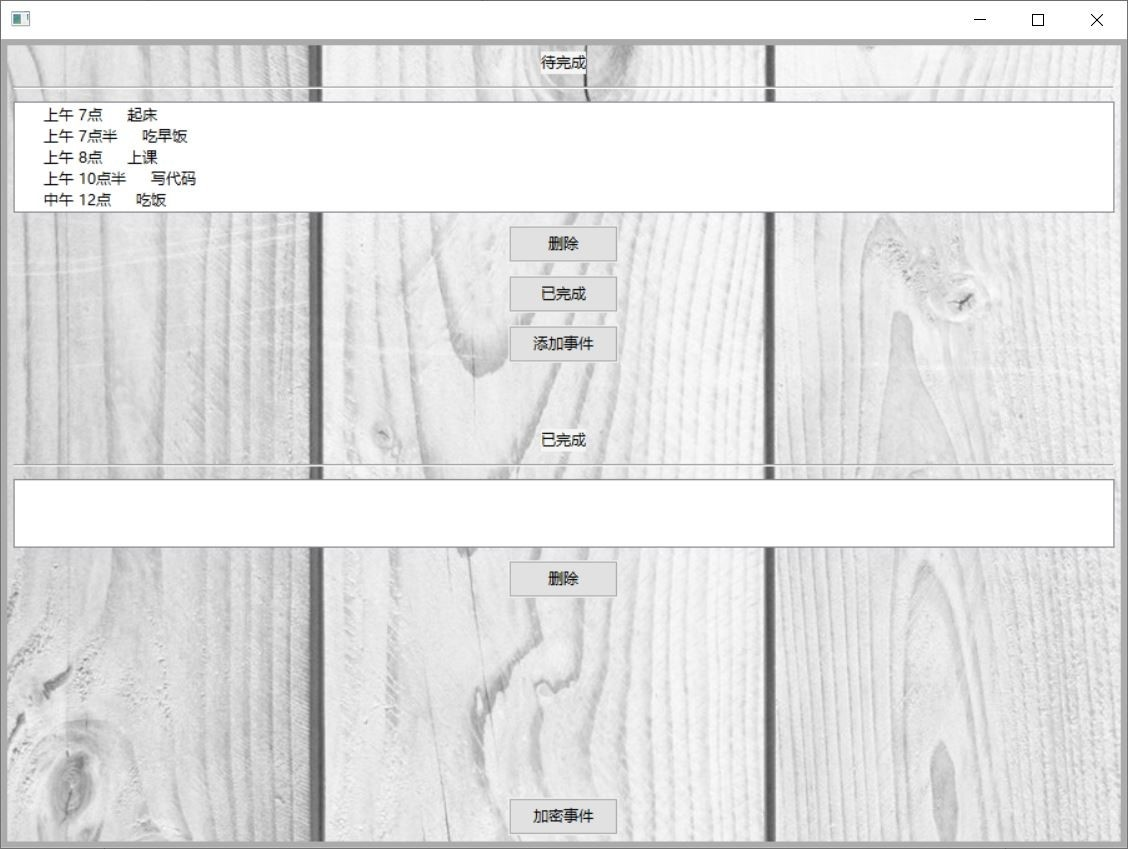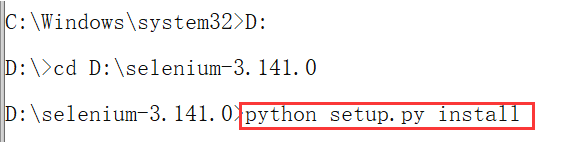2017-11-26 17:20:24 u014253445 阅读数 11716
• ###### python数据分析与机器学习实战【2019新版】

购买课程后，请扫码进入学习群，获取唐宇迪老师答疑 Python数据分析与机器学习实战教程，该课程精心挑选真实的数据集为案例，通过python数据科学库numpy,pandas,matplot结合机器学习库scikit-learn完成一些列的机器学习案例。课程以实战为基础，所有课时都结合代码演示如何使用这些python库来完成一个真实的数据案例。算法与项目相结合，选择经典kaggle项目，从数据预处理开始一步步代码实战带大家入门机器学习。学完该课程即可： 1.掌握Python数据科学工具包，包括矩阵数据处理与可视化展示。 2.掌握机器学习算法原理推导，从数学上理解算法是怎么来的以及其中涉及的细节。 3.掌握每一个算法所涉及的参数，详解其中每一步对结果的影响。 4.熟练使用Python进行建模实战，基于真实数据集展开分析，一步步完成整个建模实战任务。

93944 人正在学习 去看看 唐宇迪

# python数据挖掘项目实战记录

1. 数据预处理方法
2. 建立模型方法
3. 绘制图形

## （一）数据预处理的方法

### 数据读取

``````#SVM，bayes，ANN，D_Tree，等需要操作的数据是矩阵，需要
data=data.as_matrix() #将series数据转为矩阵形式的训练集

from numpy.random import shuffle
shuffle(data) #随机打乱数据
x_train=data_train[:,2:]*30 #将特征放大

import pickle
pickle.dump(model, open('../tmp/svm.model', 'wb'))# 保存模型

#将数据保存为固定文件格式
pd.DataFrame(cm_train, index=range(5),columns=range(5)).to_excel(outputfile1)
``````

### 一、拉格朗日插值法

``````from scipy.interpolate import lagrange
# 取数，两个列表尾接
y = s[list(range(n - k, n)) + list(range(n + 1, n + 1 + k))]
y = y[y.notnull()]
res=lagrange(y.index, list(y))(n)   #输入索引，和该列数据 n为空值下标``````

### 二、series的内置插值法

``````df = pd.DataFrame(data, columns=[0, 1, 2])
df.interpolate()``````

### 四、数据探索describe(include=’all).T

``````explore = data.describe(include='all').T
explore['null'] = len(data) - explore['count']
explore = explore[['null', 'max', 'min', 'std']]# count,unique,top,freq,mean,std,min,50%,max等选取几个属性

#计算相关系数矩阵，data必须是n*n矩阵
print(np.round(data.corr(method='pearson'), 2))
``````

### 五、数据清洗

``````data = data[data['SUM_YR_1'].notnull() & data['SUM_YR_2'].notnull()]
index1 = data['SUM_YR_1'] != 0
index2 = data['SUM_YR_2'] != 0
index3 = (data['SEG_KM_SUM'] == 0) & (data['avg_discount'] == 0)
data = data[index1 | index2 | index3]
``````

### 六、属性转换

``````
# 选取某列
data=data[data['TARGET_ID']==184].copy()  #获取该条件下的数据的副本
data_group=data.groupby('COLLECTTIME')  #以时间分组

def attr_trans(x):  # 定义属性变换函数
#创建新的series
result=pd.Series(index=['SYS_NAME', 'CWXT_DB:184:C:\\', 'CWXT_DB:184:D:\\', 'COLLECTTIME'])  #设置列标
result['SYS_NAME'] = x['SYS_NAME'].iloc #获取该属性值，唯一
result['COLLECTTIME'] = x['COLLECTTIME'].iloc #获取该属性值，唯一
result['CWXT_DB:184:C:\\'] = x['VALUE'].iloc #获取属性值A
result['CWXT_DB:184:D:\\'] = x['VALUE'].iloc #获取属性值B，等等
return result

data_processed = data_group.apply(attr_trans)  # 逐组处理
``````

### 七、数据归一化和标准化

``data = (data - data.mean(axis=0)) / data.std(axis=0)  # 按列选取均值和标准差。矩阵操作``

``````data=(data-data.min())/(data.max()-data.min())
data=data.reset_index()
``````

### 八、数据离散化

``````    for i in range(len(keys)):
# 调用k-means算法，进行聚类离散化
r1 = pd.DataFrame(kmodel.cluster_centers_, columns=[typelabel[keys[i]]])  # 聚类中心,A
r2 = pd.Series(kmodel.labels_).value_counts()  # 分类统计
r2 = pd.DataFrame(r2, columns=[typelabel[keys[i]] + 'n'])  #统计量, An

r = pd.DataFrame(pd.concat([r1, r2], axis=1))  #聚类中心与类别数目匹配连接
r = r.sort_values(typelabel[keys[i]])
r.index = [1, 2, 3, 4]

r[typelabel[keys[i]]] = pd.rolling_mean(r[typelabel[keys[i]]], 2)  # rolling_mean()用来计算相邻2列的均值，以此作为边界点。
r[typelabel[keys[i]]] = 0.0  # 这两句代码将原来的聚类中心改为边界点。
result = result.append(r.T) #转置添加

result = result.sort_index()  # 以Index(A,B,C,D,E,F)顺序排序，保存
result.to_excel(processedfile)
``````

### 九、图像切割和颜色矩阵提取

1. 一阶颜色矩：采用一阶原点矩，反应图像的整体明暗程度
Ei=1/N * ∑(j:1-N) Pij
2. 二阶颜色矩：反应图像颜色的分布范围
σi=(1/N *∑j:1-N (Pij-Ei)^2) ^1/2
3. 三阶颜色矩：反应图像颜色分布的对称性

### 十一、行为分析与服务推荐

#### 推荐

``````# 基于物品的协同过滤算法
def Jaccard(a, b):
return 1.0 * (a * b).sum() / (a + b - a * b).sum()
class Recommender():
sim = None
def similarity(self, x, distance):
y = np.ones((len(x), len(x)))
for i in range(len(x)):
for j in range(len(x)):
y[i, j] = distance(x[i], x[j])
return y
def fit(self, x, distance=Jaccard):  #x传入的是矩阵（行：物品，列：用户）
self.sim = self.similarity(x, distance) #计算相似度
def recommend(self, a): #传入预测用户的购买记录的矩阵.T = n * 1
return np.dot(self.sim, a) * (1 - a)``````

### 十三、文本预处理

``````#数据去重
l1 = len(data)
data = pd.DataFrame(data.unique()) #选取数据列进行unique()
l2 = len(data)
data.to_csv(outputfile, index = False, header = False, encoding = 'utf-8')
print(u'删除了%s条评论。' %(l1 - l2))

#机械压缩去词，去除连续重复语料，和短句子删除过滤较多垃圾信息

#文本评论分词
mycut = lambda s: ' '.join(jieba.cut(s)) #自定义简单分词函数
data1 = data1.apply(mycut)#对于读入的数据执行分词函数
data2 = data2.apply(mycut)#通过“广播”形式分词，加快速度。

#先将文本正负面评价分开，然后再进行LDA主题分析。COSTCM6中的情感分析做及其分类，生成正面情感和负面情感

# 正面主题分析
from gensim import corpora, models
pos_dict = corpora.Dictionary(pos)
pos_corpus = [pos_dict.doc2bow(i) for i in pos]
pos_lda = models.LdaModel(pos_corpus, num_topics=3, id2word=pos_dict)
for i in range(3):
neg_lda.print_topic(i)  # 输出每个主题
``````

## （二）、模型方法

### 一、LM神经网络

API:

``````from keras.models import Sequential
from keras.layers import Dense, Activation
net = Sequential()
net.fit(train[:, :3], train[:, 3], epochs=1000, batch_size=1)#传入的是矩阵，读取excel需要把数据.as_matrix()
net.save_weights(netfile)
predict_result = net.predict_classes(train[:, :3]).reshape(len(train))``````

### 二、CART决策树

API：
fit(); predict();

``````# 构建CART决策树模型
from sklearn.tree import DecisionTreeClassifier
tree = DecisionTreeClassifier()
tree.fit(train[:, :3], train[:, 3])
plt = cm_plot(test[:, 3], tree.predict(test[:, :3]))#获取结果
plt.show()``````

### 三、K-Means K聚类算法

``````from sklearn.cluster import KMeans
import pandas as pd
inputFile = '../data/zscoreddata.xls'
kmodel = KMeans(n_clusters=5, n_jobs=4)
kmodel.fit(data)
print(kmodel.cluster_centers_)``````

### 四、SVM支持向量机

``````from sklearn import svm
smodel=svm.SVC()  #建立模型
smodel.fit(x_train,y_train)  #训练模型
res=smodel.predict(x_test)  #预测测试集
``````

## （三）、绘制图形

ROC曲线：分类方法的性能

### 混淆矩阵

recall=TP/(TP+FN) ：表示推荐的产品，占用户喜欢产品的概率

``````from sklearn.metrics import confusion_matrix  # 导入混淆矩阵函数
cm = confusion_matrix(y, yp)  # 混淆矩阵如下
# CM [[TP,FP],[FN,TN]]
#例如['TP', 'FP', 'FN', 'TN'] == [46, 2, 7, 4]

cm_train = confusion_matrix(train_label, smodel.predict(trainSet))
cm_test = confusion_matrix(test_label, smodel.predict(testSet))
pd.DataFrame(cm_train).to_excel(outFile1)
pd.DataFrame(cm_test).to_excel(outFile2)
``````

### ROC曲线

``````from sklearn.metrics import roc_curve  # 导入ROC曲线函数
fpr, tpr, thresholds = roc_curve(test[:, 3], tree.predict_proba(test[:, :3])[:, 1], pos_label=1)
plt.plot(fpr, tpr, linewidth=2, label='ROC of CART', color='green')  # 作出ROC曲线``````

### 聚类群图

``````import matplotlib.pyplot as plt
centers = kmodel.cluster_centers_
for i in range(5):
plt.plot([2, 4, 6, 8, 10], centers[i], label='group' + str(i),marker='o')  #设置横轴纵轴分别对应5个点
plt.ylabel('values')
plt.xlabel('index: L R F M C')
plt.show()``````

### 层次聚类谱图

``````import matplotlib.pyplot as plt
#这里使用scipy的层次聚类函数

Z = linkage(data_udf, method = 'ward', metric = 'euclidean') #谱系聚类图
P = dendrogram(Z, 0) #画谱系聚类图
plt.show()
``````

python关于大数据小项目 相关内容

2019-09-25 14:54:16 S1998F 阅读数 377
• ###### python数据分析与机器学习实战【2019新版】

购买课程后，请扫码进入学习群，获取唐宇迪老师答疑 Python数据分析与机器学习实战教程，该课程精心挑选真实的数据集为案例，通过python数据科学库numpy,pandas,matplot结合机器学习库scikit-learn完成一些列的机器学习案例。课程以实战为基础，所有课时都结合代码演示如何使用这些python库来完成一个真实的数据案例。算法与项目相结合，选择经典kaggle项目，从数据预处理开始一步步代码实战带大家入门机器学习。学完该课程即可： 1.掌握Python数据科学工具包，包括矩阵数据处理与可视化展示。 2.掌握机器学习算法原理推导，从数学上理解算法是怎么来的以及其中涉及的细节。 3.掌握每一个算法所涉及的参数，详解其中每一步对结果的影响。 4.熟练使用Python进行建模实战，基于真实数据集展开分析，一步步完成整个建模实战任务。

93944 人正在学习 去看看 唐宇迪1.前端图形化界面（GUI）：wxPython + wxFromBuilder

2.后端MySQL数据库 + Python

https://github.com/supfeng/mynote

ps.如果想要打开新世界的大门，可以扫描下方的二维码，关注微信公众号「SuperFeng」。python关于大数据小项目 相关内容

2019-04-15 20:49:58 weixin_44230339 阅读数 65
• ###### python数据分析与机器学习实战【2019新版】

购买课程后，请扫码进入学习群，获取唐宇迪老师答疑 Python数据分析与机器学习实战教程，该课程精心挑选真实的数据集为案例，通过python数据科学库numpy,pandas,matplot结合机器学习库scikit-learn完成一些列的机器学习案例。课程以实战为基础，所有课时都结合代码演示如何使用这些python库来完成一个真实的数据案例。算法与项目相结合，选择经典kaggle项目，从数据预处理开始一步步代码实战带大家入门机器学习。学完该课程即可： 1.掌握Python数据科学工具包，包括矩阵数据处理与可视化展示。 2.掌握机器学习算法原理推导，从数学上理解算法是怎么来的以及其中涉及的细节。 3.掌握每一个算法所涉及的参数，详解其中每一步对结果的影响。 4.熟练使用Python进行建模实战，基于真实数据集展开分析，一步步完成整个建模实战任务。

93944 人正在学习 去看看 唐宇迪

# Python学习for数据可视化项目（一）

## 1 绘制简单的折线图

``````import matplotlib.pyplot as plt
#导入matplotlib中的pyplt模块，并指定别名plt
squares=[1,4,9,16,25]
#用pyplt模块中的plot方法对列表进行处理
plt.plot(squares)
#打开matplotlib查看器，显示绘制图像
plt.show()
``````## 2.绘制折线图与散点图

### 2.1 修改标签文字和线条粗细

plot()：可以用**linewidth**指定线条的粗细。

title()：第一个参数为图表标题内容，可用**fontsize**指定文字大小。

xlabel()：第一个参数为x轴标题，可用**fontsize**指定文字大小。

ylabel():设置y轴，同上。

tick_params():设置刻度的样式，如**axis选‘x’，’y’，‘both’来指定轴，labelsize**指定刻度数字大小。

``````import matplotlib.pyplot as plt

squares=[1,4,9,16,25]

plt.plot(squares,linewidth=5)
plt.title('Square Numbers',fontsize=24)
plt.xlabel('Value',fontsize=14)
plt.ylabel('Square of Value',fontsize=14)
plt.tick_params(axis='x',labelsize=14)
plt.show()
``````### 2.3 校正图形

``````import matplotlib.pyplot as plt

input_value=[1,2,3,4,5]
squares=[1,4,9,16,25]

plt.plot(input_value,squares,linewidth=5)
plt.title('Square Numbers',fontsize=24)
plt.xlabel('Value',fontsize=14)
plt.ylabel('Square of Value',fontsize=14)
plt.tick_params(axis='x',labelsize=14)
plt.show()
``````### 2.3 使用scatter()绘制散点图并设置其样式

``````import matplotlib.pyplot as plt

plt.scatter(2,4,s=200)
plt.title('Square Numbers',fontsize=24)
plt.xlabel('Value',fontsize=14)
plt.ylabel('Square of Value',fontsize=14)
plt.tick_params(axis='both',which='major',labelsize=14)
plt.show()
``````### 2.4 使用scatter()绘制一系列点

``````import matplotlib.pyplot as plt

x_value=[1,2,3,4,5]
y_value=[1,4,9,16,25]

plt.scatter(x_value,y_value,s=200)
plt.title('Square Numbers',fontsize=24)
plt.xlabel('Value',fontsize=14)
plt.ylabel('Square of Value',fontsize=14)
plt.tick_params(axis='both',which='major',labelsize=14)
plt.show()
``````### 2.5 自动计算数据

``````import matplotlib.pyplot as plt
x_value=list(range(1,1001))
y_value=[x**2 for x in x_value]

plt.scatter(x_value,y_value,s=20)
plt.title('Square Numbers',fontsize=24)
plt.xlabel('Value',fontsize=14)
plt.ylabel('Square of Value',fontsize=14)
plt.tick_params(axis='both',which='major',labelsize=14)

plt.axis([0,1100,0,1100000])#设置每个坐标轴的取值范围
plt.show()
``````### 2.6 删除数据点的轮廓

matplotlib默认散点图为蓝色点和黑色轮廓。

``````plt.scatter(x_value,y_value,edgecolors='none',s=20)
``````

### 2.7 自定义颜色

``````plt.scatter(x_value,y_value,c='red',edgecolor='none',s=20)
``````

c参数也可以用RGB颜色模式来自定义颜色。向其传递一个有3个元素的元组。元素值在0~1之间，分别表示红色、绿色、蓝色的分量，值越接近0颜色越深，反之越浅。

``````plt.scatter(x_value,y_value,c=(0,0,0.8),edgecolor='none',s=20)
``````

### 2.8 使用颜色映射

**颜色映射（colormap）**是一系列颜色，它们从其实颜色渐变到结束颜色。在可视化中，颜色映射用于突出数据的规律。如可以用较浅的颜色来显示较小的值，用较深的颜色来显示较大的值。

``````#将c设置成一个y值列表，参数cmap设置映射的颜色
plt.scatter(x_values,y_value,c=y_value,cmap=plt.cm.Blues,edgecolor='none',s=20)
``````### 2.9 自动保存图标

``````plt.savefig('squares_plt.png',bbox_inches='tight')
``````

## 3 随机漫步

### 3.1 创建RandomWalk()类

``````from random import choice

class RandomWalk():
'''一个生成随机漫步数据的类'''
def __init__(self,num_points=5000):
'''初始化属性'''
self.num_points=num_points

#所有随机漫步都始于（0，0）
self.x_values=
self.y_values=
``````

### 3.2 选择方向

``````    def fill_walk(self):
'''计算生成随机漫步的点'''
#不断生成数据直到达到指定的长度
while len(self.x_values)<self.num_points:
#漫步方向，距离，实际步长
x_direction=choice([1,-1])
x_distance=choice([0,1,2,3,4])
x_step=x_direction*x_distance

y_direction=choice([1,-1])
y_distance=choice([0,1,2,3,4])
y_step=y_direction*y_distance
#若原地踏步则重新生成
if x_step==0 and y_step==0:
continue
#下一步将从上一步出发，所以将步长加上列表的最后一个值
next_x=self.x_values[-1]+x_step
next_y=self.y_values[-1]+y_step
#将下一步的坐标加入列表
self.x_values.append(next_x)
self.y_values.append(next_y)
``````

### 3.3 绘制随机漫步图

``````import matplotlib.pyplot as plt
from random_walk import RandomWalk

rw=RandomWalk()
rw.fill_walk()
plt.scatter(rw.x_values,rw.y_values,s=15)
plt.show()
``````### 3.4 模拟多次随机漫步

``````import matplotlib.pyplot as plt
from random_walk import RandomWalk

while True:
rw=RandomWalk()
rw.fill_walk()
plt.scatter(rw.x_values,rw.y_values,s=15)
plt.show()

keep_running=input('Make another walk?(y/n):')
if keep_running=='n':
break
``````

### 3.5 给点着色

``````import matplotlib.pyplot as plt
from random_walk import RandomWalk

while True:
rw=RandomWalk()
rw.fill_walk()
#使用list生成一个与点总数相同的列表
point_numbers=list(range(rw.num_points))
plt.scatter(rw.x_values,rw.y_values,c=point_numbers,
cmap=plt.cm.Blues,edgecolors='none',s=15)
plt.show()

keep_running=input('Make another walk?(y/n):')
if keep_running=='n':
break
``````### 3.6 重新绘制起点和终点

``````--sinp--
#突出起点和重点
plt.scatter(0,0,c='green',edgecolor='none',s=100)
plt.scatter(rw.x_value[-1],rw.y_value[-1],c='red',edgecolor='none',s=100)
--sinp--
``````

### 3.7 隐藏坐标轴

``````	#隐藏坐标轴
plt.axes().get_xaxis().set_visible(False)
plt.axes().get_yaxis().set_visible(False)
``````### 3.8 增加点数

``````--sinp--
#设置50000个点
rw=RandomWalk(50000)
--Sinp--
#每个点大小调整为1
plt.scatter(rw.x_value,rw.y_value,c=points_numbers,
cmap=plt.cm.Blues,edgecolor='none',s=1)
--sinp--
``````### 3.9 调整尺寸以适合屏幕

**figure()用于指定图表的宽度、高度、分辨率和背景色。可为其形参figsize**指定一个元组，来设定绘图窗口的大小，单位为英寸。

``````plt.figure(figsize=(10,6))
``````

``````plt.figure(dpi=28,figsize=(10,6))
``````

## 4 使用Pygal模拟掷骰子

### 4.1 创建Die类

``````from random import randint

class Die():
#将骰子默认设为6面
def __init__(self,num_sides=6):
self.num_sides=num_sides
#模拟掷骰子，随机生成1~6中的数
def roll(self):
return  randint(1,self.num_sides)
``````

### 4.2 掷骰子

``````from die import Die

D6=Die()
results=[]
for roll_num in range(100):
result=D6.roll()
results.append(result)

print(results)
``````

``````[1, 5, 4, 4, 6, 3, 6, 5, 2, 1, 1, 3, 1, 3, 2, 6, 5, 2, 3, 2, 1, 5, 3, 5, 4, 4, 4, 1, 3, 4, 1, 4, 3, 4, 6, 3, 6, 1, 1, 2, 4, 5, 3, 3, 3, 5, 3, 5, 1, 1, 5, 6, 5, 2, 5, 4, 2, 2, 1, 1, 6, 5, 1, 4, 5, 3, 6, 5, 5, 5, 5, 4, 3, 1, 1, 3, 6, 5, 4, 4, 6, 6, 1, 2, 3, 6, 6, 2, 4, 5, 6, 2, 2, 5, 3, 6, 5, 3, 6, 2]
``````

### 4.3 分析结果

``````#此部分与上相同，可将掷骰子次数提升到1000
#这里的die是骰子类的模块名，后文不表
from die import Die

D6=Die()
results=[]
for roll_num in range(1000):
result=D6.roll()
results.append(result)
print(results)
#创建一个列表来统计1~6出现的次数
frequencies=[]
for value in range(1,D6.num_sides+1):
frequencie=results.count(value)
frequencies.append(frequencie)

print(frequencies)
``````

### 4.4 绘制直方图

``````import pygal

--接上小节代码--

hist=pygal.Bar()
#指定直方图标题
hist.title='Results of rolling one D6 1000 times.'
#指定直方图x轴标签
hist.x_labels=['1','2','3','4','5','6']
#指定直方图x方向标题
hist.x_title='Results'
#指定直方图y方向标题
hist.y_title='Frequency of Result'

#指定直方图的数据名称，和数据组成的列表
#保存直方图文件，并以参数的字符串命名
hist.render_to_file('die_visual.svg')
``````### 4.5 同时掷两个骰子

``````import pygal
from die import Die
#创建两个骰子实例
d6_1=Die()
d6_2=Die()
results=[]
for roll_num in range(1000):
#点数相加
result=d6_1.roll()+d6_2.roll()
results.append(result)

print(results)

frequencies=[]
max_result=d6_1.num_sides+d6_2.num_sides
#修改总点数范围
for value in range(2,max_result+1):
frequencie=results.count(value)
frequencies.append(frequencie)

print(frequencies)

hist=pygal.Bar()
hist.title='Results of rolling one D6 1000 times.'
#修改直方图横坐标标签
hist.x_labels=['2','3','4','5','6','7','8','9','10','11','12']
hist.x_title='Results'
hist.y_title='Frequency of Result'
#修改直方图数据名称
#修改直方图文件保存名称，否则会覆盖原图
hist.render_to_file('dice_visual.svg')
``````### 4.6 同时掷两个面数不同的骰子

``````import pygal
from die import Die

d6_1=Die()
#将第二个骰子面数设为10
d10=Die(10)
results=[]
#掷50000次
for roll_num in range(50000):
result=d6_1.roll()+d10.roll()
results.append(result)

print(results)

frequencies=[]
max_result=d6_1.num_sides+d10.num_sides
for value in range(2,max_result+1):
frequencie=results.count(value)
frequencies.append(frequencie)

print(frequencies)
#在以下代码中做出相应修改
hist=pygal.Bar()
hist.title='Results of rolling one D6 and one D10 50000 times.'
hist.x_labels=['2','3','4','5','6','7','8','9','10','11','12','13','14','15','16']
hist.x_title='Results'
hist.y_title='Frequency of Result'

hist.render_to_file('different_dice.svg')
``````python关于大数据小项目 相关内容

2019-06-11 18:48:28 qq_39394264 阅读数 285
• ###### python数据分析与机器学习实战【2019新版】

购买课程后，请扫码进入学习群，获取唐宇迪老师答疑 Python数据分析与机器学习实战教程，该课程精心挑选真实的数据集为案例，通过python数据科学库numpy,pandas,matplot结合机器学习库scikit-learn完成一些列的机器学习案例。课程以实战为基础，所有课时都结合代码演示如何使用这些python库来完成一个真实的数据案例。算法与项目相结合，选择经典kaggle项目，从数据预处理开始一步步代码实战带大家入门机器学习。学完该课程即可： 1.掌握Python数据科学工具包，包括矩阵数据处理与可视化展示。 2.掌握机器学习算法原理推导，从数学上理解算法是怎么来的以及其中涉及的细节。 3.掌握每一个算法所涉及的参数，详解其中每一步对结果的影响。 4.熟练使用Python进行建模实战，基于真实数据集展开分析，一步步完成整个建模实战任务。

93944 人正在学习 去看看 唐宇迪

# Python小项目：爬虫

## 一、环境搭建

1. 浏览器：FireFox_v66.0.5_x64.zip（火狐浏览器） 提取码：2zk0

2. 三方库selenium：selenium-3.141.0.tar.gz 提取码：anq9

3. 与浏览器版本对应的webdriver：geckodriver-v0.23.0-win64.zip 提取码：z02m4. 安装方式：
a. 在线安装：pip install packageName
b. 离线安装：
c. 卸载：pip uninstall packageName
d. 查看三方库的安装情况：pip list

## 二、离线安装selenium

1. 解压安装包

2. 目录切换到解压文件夹

`cd D:\selenium-3.141.0`

3. 安装
python setup.py install

## 三、配置webdriver

1. 解压
geckodriver.exe

2. 移动到指定文件夹下
D:\Anaconda3\Scripts

3. 代码

`````` # 从selenium库中导入浏览器驱动模块
from selenium import webdriver
# 实例化浏览器驱动模块下对应的浏览器
# browser = webdriver.Firefox()
browser = webdriver.PhantomJS
# 最大化窗口
browser.maximize_window()
# 打开浏览器
browser.get('http://www.baidu.com')

# 进行页面操作
# 方法一：在浏览器中找到搜索框对应的id属性，并发送数值
#browser.find_element_by_id('kw').send_keys('Python')

# 方法二：name属性
#browser.find_element_by_name('wd').send_keys('Python')

# 方法三：class属性
#browser.find_element_by_class_name('s_ipt').send_keys('Python')

# 方法四：xpath
browser.find_element_by_xpath('//*[@id="kw"]').send_keys('Python')
``````

##在页面上进行操作：

``````		# 1.发送数据send_keys(),
# 2.清空数据clear()，
browser.find_element_by_xpath('//*[@id="kw"]').clear()
# 3.点击
browser.find_element_by_xpath('//*[@id="kw"]').click()
# 4.打印页面文本信息text
#page_source:页面源码
print(browser.page_source)
# 退出浏览器
#browser.quit()
``````

## 四、练习

1. 从中找到：IP端口号 协议类型

2. 验证ip和端口号是否可用，并且把是否可用的结果进行记录

3. 20组数据

`````` from selenium import webdriver
from telnetlib import Telnet
from openpyxl import Workbook

browser = webdriver.Firefox()
url = 'http://www.xicidaili.com/nn'
browser.get(url)

wb = Workbook()
active = wb.active
active.title = 'ip信息'
active.append(['IP', 'Port', 'Pro', 'Connection'])
for t in range(2,102):
xcIP_1 = browser.find_element_by_xpath('/html/body/div/div/table/tbody/tr[%s]/td'%t).text
xcPort_1 = browser.find_element_by_xpath('/html/body/div/div/table/tbody/tr[%s]/td'%t).text
xcPro_1 = browser.find_element_by_xpath('/html/body/div/div/table/tbody/tr[%s]/td'%t).text
# print(xcIP_1,xcPort_1,xcPro_1)

try:
Telnet(xcIP_1,xcPort_1,timeout=0.5)
Connection = 'Success'
except:
Connection = 'Failed'

active.cell(row=t, column=1, value=xcIP_1)
active.cell(row=t, column=2, value=xcPort_1)
active.cell(row=t, column=3, value=xcPro_1)
active.cell(row = t,column = 4,value = Connection)
wb.save('./ip1.xlsx')
``````

--->有问题请联系QQ1436281495^_^

python关于大数据小项目 相关内容

2019-11-20 17:16:05 u014639984 阅读数 12
• ###### python数据分析与机器学习实战【2019新版】

购买课程后，请扫码进入学习群，获取唐宇迪老师答疑 Python数据分析与机器学习实战教程，该课程精心挑选真实的数据集为案例，通过python数据科学库numpy,pandas,matplot结合机器学习库scikit-learn完成一些列的机器学习案例。课程以实战为基础，所有课时都结合代码演示如何使用这些python库来完成一个真实的数据案例。算法与项目相结合，选择经典kaggle项目，从数据预处理开始一步步代码实战带大家入门机器学习。学完该课程即可： 1.掌握Python数据科学工具包，包括矩阵数据处理与可视化展示。 2.掌握机器学习算法原理推导，从数学上理解算法是怎么来的以及其中涉及的细节。 3.掌握每一个算法所涉及的参数，详解其中每一步对结果的影响。 4.熟练使用Python进行建模实战，基于真实数据集展开分析，一步步完成整个建模实战任务。

93944 人正在学习 去看看 唐宇迪

### python一些练手小项目

Pycharm+django2.2+python3.6+MySQL实现简单的考试报名系统
Pycharm+Django之使用模型
django基础之数据库操作

python关于大数据小项目 相关内容